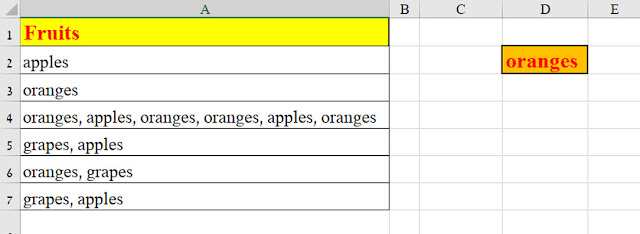# Excel – How many times does a string appear in a cell

Suppose you want to find out how many times the word “oranges” appears in cell A4Pic 1: we want to search the string oranges within cell A4

The simplest method would be with the SUBSTITUTE function.

Here is a formula that can be seen, for example, on the Exceljet.net website:

and, after adaptation to our case:

Pic 2: the “traditional” method is not flexible…

But what if want to find out not just the number of occurrences of the substring “oranges” within the cell, but its number of occurrences starting from a certain location within the cell?

For example, starting from the 10th character?
The formula displayed above isn’t flexible enough for the answer.

So, I found a more flexible solution using the a-m-a-z-i-n-g SEQUENCE function,

As shown in this picture:

Pic 3: my method enables flexibility: start searching from any position

So, the formula in C8, counts the number of “oranges” in cell A4, but only from the position stated in the parameter: 10

=SUM(IF(ISNUMBER(FIND(D2,MID(A4,SEQUENCE(LEN(A4),,F2),LEN(D2)))),1,0))

Neat, isn’t it?